Algorithms

4K

Solutions

4K

Contributors

An algorithm is a self-contained step-by-step set of operations to be performed. Algorithms exist that perform calculation, data processing, and automated reasoning. Starting from an initial state and initial input (perhaps empty), the instructions describe a computation that, when executed, proceeds through a finite number of well-defined successive states, eventually producing "output" and terminating at a final ending state. The transition from one state to the next is not necessarily deterministic; some algorithms, known as randomized algorithms, incorporate random input.

Share tech news, updates, or what's on your mind.
Example of a Neural Network?

I am interested to know what I can do with Neural Networks and hope to see an example.

Got any?

Thanks.
0
Hello,
I get the number of minutes from a database.
The number of minutes should be displayed as a line in a coordinate system.
For a minute, I take a pixel.
But if I want to display 20 hours, then the line is too long.
Is there a way to display the minutes exactly by scaling down or up (without having to round)?

Is it possible to display for a minute 0.5 pixel?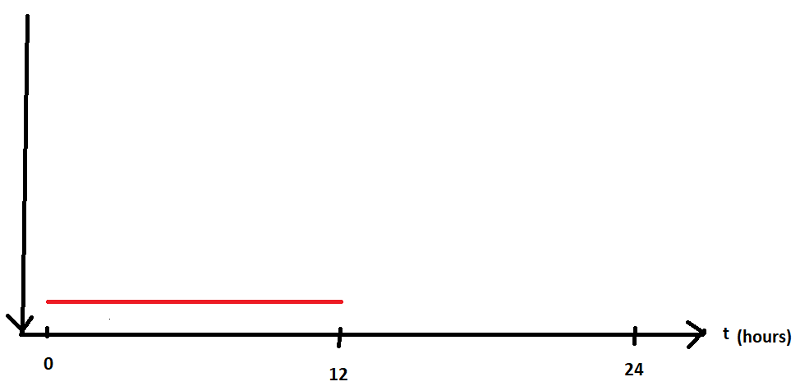Thank you
0
Hello,
I have on my program several rectangles. The rectangles are moveable.  But Collisions should be avoided when moving.
For example:
When I move the blue rectangle on the red rectangle then a collision happened.
The blue rectangle should then be placed under the red rectangle.
But then a collision with the green rectangle happens.
So rectangle blue should be placed between rectangle green and red.
I draw a rectangle after mouseUp Event.

How can I solve this problem?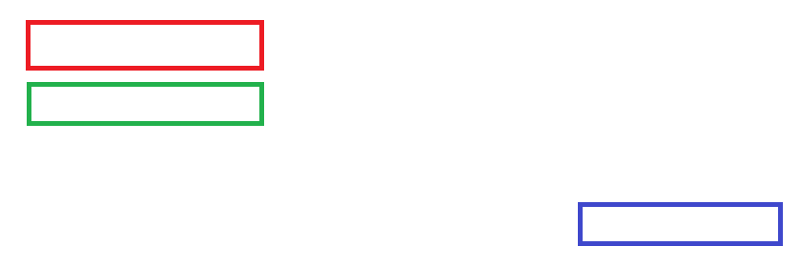Result: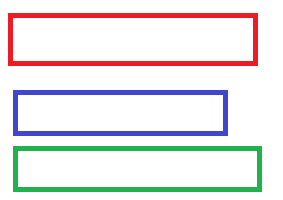Thank you
0
I'm trying to figure out an algorithm for the following problem:

- There should always be two rectangles connected to a line
- The lines always run from the middle of the rectangle1 to the center of the rectangle2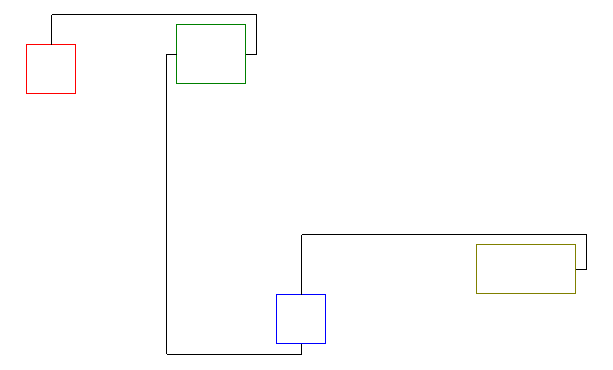- One rectangle has two inputs and two outputs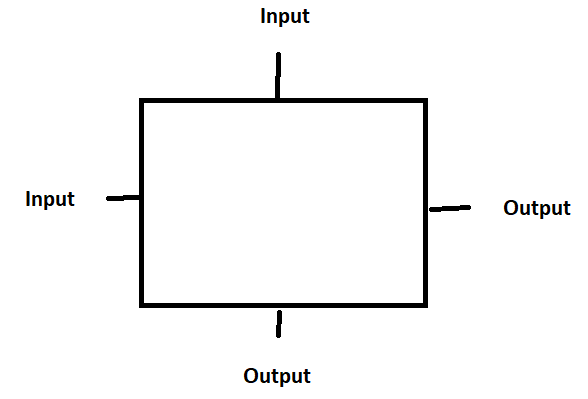- An output can only be connected to one input
- An input can only be connected to one output
- The first rectangle has a connection from the output to the input
- You can move the rectangles as you like
- All lines must be outside the rectangles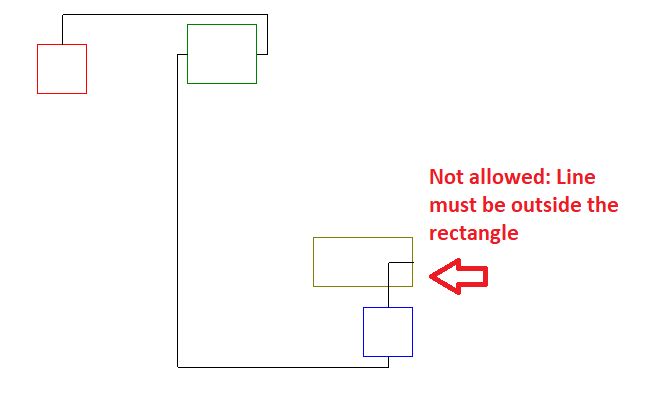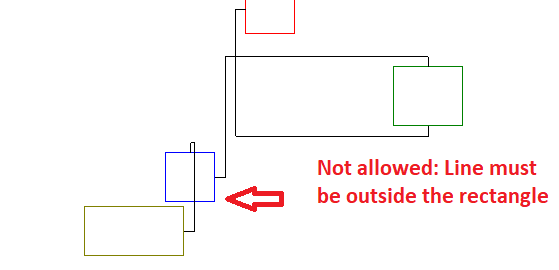- The lines may intersect

My idea is to always compare two rectangles. The left and the right rectangle.
I first determine the left rectangle of the two rectangles.
Then I ask if the left rectangle is above or below the right rectangle and draw the lines accordingly

In addition, I still have to query whether the center of the smaller rectangle is within the larger rectangle and draw the lines, then different.

My questions:
1) Is this algorithm feasible under these conditions?
2) What else do I have to keep in mind about my algorithm, or do you have ideas for a better algorithm?

I am very grateful for numerous tips
0
Can someone explain to me where consensus algorithms fit within the TCP/IP model?  Let use Raft as an example.  Does it use multicast?  Does it use it's own transport number or TCP/UDP?  Does it live on the application layer?

Thanks
0
Request help from the experts to suggest the best name matching algorithm, logic description and code to reuse for executing name matching function - java or excel can do..
0
Dual pipe producer/consumer blocked by read.

The issue is in the producer:
prevents the producer from working because the consumer has yet to write anything, how can I avoid this?

#include <unistd.h>
#include <stdio.h>
#include <stdlib.h>
#define SIZE 10

int shared_arr[SIZE];
int cnt = 0, in = 0, out = 0;
int consumer_to_producer, producer_to_consumer;

void consumer();
void producer();

int main() {
pipe(consumer_to_producer); // consumer to producer
pipe(producer_to_consumer); // producer to consumer

if (fork() == 0) {
/* child process */
consumer();
} else {
/* parent process */
producer();
sleep(3);
}

exit(0);
}

void consumer() {
/* consumer process */
close(producer_to_consumer); // Close write end, we don't need it
close(consumer_to_producer); // This fcn doesn't need read end
while (1) {
/* if buffer is full, consume it */
// read in cnt from producer so we can check if it's full

if (cnt == SIZE) { /* If full, consume */
printf("I am consuming\t%d\t%d\n", shared_arr[in], out);
out = (out + 1) % SIZE;
cnt--;
}

0
All,

I'm looking to find a way to visualize in a very clear way the age of project tasks. What I have in mind is some sort of bubble chart or distribution of points (that can be shown on a web page) where each point or bubble takes on a certain color based on the time since it was last modified. If possible I would also like to group these points based on the project they are contained within, the person responsible for executing the task or some other type of relationship or tagging scheme.

Over time and as the number of tasks increase it would be easy to see how we are doing overall simply based on the predominant colors on the chart. A lot of green or green-ish colors would mean we are doing fine and are keeping up with all of our projects and a lot of red would mean that a lot of tasks have been left unattended for a long time.

Since this is totally new territory for me I am simply looking for some good advice and pointers on where to start my research. Are the good software to use for this? Are there any special algorithms or other theoretical concepts I should read up on and so forth.

Any advice will be greatly appreciated.

Best regards
Daniel Södling
0
Hi
I am working on GIS applications and found the slope algorith in the net :

ftp://ftp.ecn.purdue.edu/jshan/86/help/html/gmd/slope_percent.htm

attached is an example I used to apply the above algorithm for calculating slopes from a DEM. I am not good in math so I got two results.
Can you help me please . I got 73, -33, 56.648 and I do not how to do the arctan.
slope.doc
slope.doc
0

Algorithms

4K

Solutions

4K

Contributors

An algorithm is a self-contained step-by-step set of operations to be performed. Algorithms exist that perform calculation, data processing, and automated reasoning. Starting from an initial state and initial input (perhaps empty), the instructions describe a computation that, when executed, proceeds through a finite number of well-defined successive states, eventually producing "output" and terminating at a final ending state. The transition from one state to the next is not necessarily deterministic; some algorithms, known as randomized algorithms, incorporate random input.

Top Experts In
Algorithms
Monthly